# Minimax statistical procedure

One of the versions of optimality in mathematical statistics, according to which a statistical procedure is pronounced optimal in the minimax sense if it minimizes the maximal risk. In terms of decision functions (cf. Decision function) the notion of a minimax statistical procedure is defined as follows. Let a random variabletake values in a sampling space,, and let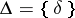be the class of decision functions which are used to make a decisionfrom the decision spaceon the basis of a realization of, that is,. In this connection, the loss function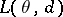, defined on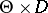, is assumed given. In such a case a statistical procedure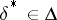is called a minimax procedure in the problem of making a statistical decision relative to the loss functionif for all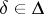,where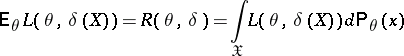is the risk function associated to the statistical procedure (decision rule); the decisioncorresponding to an observationand the minimax procedure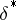is called the minimax decision. Since the quantity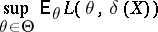shows the expected loss under the procedure,being maximal means that ifis used to choose a decisionfrom, then the largest expected risk,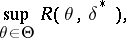will be as small as possible.Figure: m063970a

The minimax principle for a statistical procedure does not always lead to a reasonable conclusion (see Fig. a); in this case one must be guided byand not by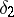, although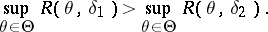The notion of a minimax statistical procedure is useful in problems of statistical decision making in the absence of a priori information regarding.